# YruRU Method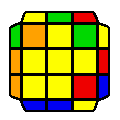##### (U2) R' U2 R U R U R2 U R2 U' R2 U R U' R U' R'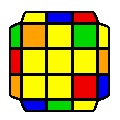##### (U') R' U2 R2 U R2 U R U' R U' R'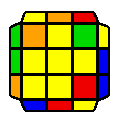##### (U') R' U2 R2 U R U R U' R' U' R2 U R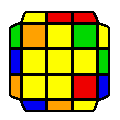##### (U') R2 U' R' U' R2 U R U R2 U R' U R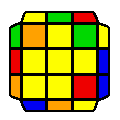##### (U') R2 U R' U' R' U' R U2 R' U' R2 U' R2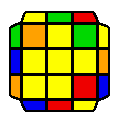##### (U') R2 U R U' R' U' R U2 R U' R2 U' R2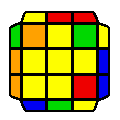##### R U R' U R U2 R'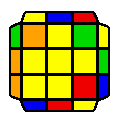##### (U') R' U2 R2 U R U' R' U R U R2 U' R'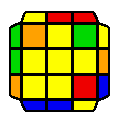##### (U') R' U2 R U R' U R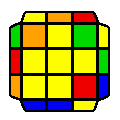##### "R U R2 U' R2 U' R2 U2 R2 U2 R'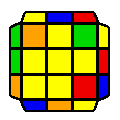##### (U') R2 U' R2 U' R' U2 R U' R' U' R' U R2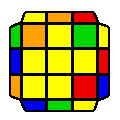##### (U') R2 U' R2 U' R U2 R U' R' U' R U R2

Average movecount: 11.8

Average double turns: 3.6

Algorithms: 12

There are two cases with 3 1x2 blocks, these are the simple sune cases.

If there is a large 2x2 block, it is a pure twist case. If in its place there is a 1x2 block but no other blocks (2 cases), then we have a cyclic shift of the bruno algorithm.

If there are no blocks (3 cases), then we either have an H-perm pattern in the 2x2 block, or depending on where the the opposites on the 2x2 block are, we have to do an algorithm that starts and ends with an R2.

There is one case with 2 blocks opposite each other. All other cases (three cases) have one block in locations other than the 2x2, and all these algorithms start with an R2.

Note, these rules are only to make learning of these cases simpler. For speed, a better recognition system is to be adopted.